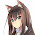## Friday, March 27, 2015

### Algebraic side effects

Haskell differentiates itself from most other functional languages by letting you reason mathematically about programs with side effects. This post begins with a pure Haskell example that obeys algebraic equations and then generalizes the example to impure code that still obeys the same equations.

#### Algebra

In school, you probably learned algebraic rules like this one:

``f * (xs + ys) = (f * xs) + (f * ys)``

Now let's make the following substitutions:

• Replace the mathematical multiplication with Haskell's `map` function
• Replace the mathematical addition with Haskell's `++` operator

These two substitutions produce the following Haskell equation:

``map f (xs ++ ys) = (map f xs) ++ (map f ys)``

In other words, if you concatenate the list `xs` with the list `ys` and then `map` a function named `f` over the combined list, the result is indistinguishable from `map`ping `f` over each list individually and then concatenating them.

Let's test this equation out using the Haskell REPL:

``````>>> map (+ 1) ([2, 3] ++ [4, 5])
[3,4,5,6]
>>> (map (+ 1) [2, 3]) ++ (map (+ 1) [4, 5])
[3,4,5,6]``````

#### Evaluation order

However, the above equation does not hold in most other languages. These other languages use function evaluation to trigger side effects, and therefore if you change the order of evaluation you change the order of side effects.

Let's use Scala to illustrate this. Given the following definitions:

``````>>> def xs() = { print("!"); Seq(1, 2) }
>>> def ys() = { print("?"); Seq(3, 4) }
>>> def f(x : Int) = { print("*"); x + 1 }``````

.. the order of side effects differs depending on whether I concatenate or map first:

``````>>> (xs() ++ ys()).map(f)
!?****res0: Seq[Int] = List(2, 3, 4, 5)
>>> (xs().map(f)) ++ (ys().map(f))
!**?**res1: Seq[Int] = List(2, 3, 4, 5)``````

One line #1, the two lists are evaluated first, printing `"!"` and `"?"`, followed by evaluating the function `f` on all four elements, printing `"*"` four times. On line #2, we call `f` on each element of `xs` before beginning to evaluate `ys`. Since evaluation order matters in Scala we get two different programs which print the punctuation characters in different order.

#### The solution

Haskell, on the other hand, strictly separates evaluation order from side effect order using a two-phase system. In the first phase you evaluate your program to build an abstract syntax tree of side effects. In the second phase the Haskell runtime executes the tree by interpreting it for its side effects. This phase distinction ensures that algebraic laws continue to behave even in the presence of side effects.

To illustrate this, we'll generalize our original Haskell code to interleave side effects with list elements and show that it still obeys the same algebraic properties as before. The only difference from before is that we will:

• Generalize pure lists to their impure analog, `ListT`
• Generalize functions to impure functions that wrap side effects with `lift`
• Generalize `(++)` (list concatenation) to `(<|>)` (`ListT` concatenation)
• Generalize `map` to `(=<<)`, which streams elements through an impure function

This means that our new equation becomes:

``````-- f  *  (xs  +  ys) = (f  *  xs)  +  (f  * ys)
f =<< (xs <|> ys) = (f =<< xs) <|> (f
=<< ys)``````

You can read this as saying: if we concatenate `xs` and `ys` and then stream their values through the impure function `f`, the behavior is indistinguishable from streaming each individual list through `f` first and then concatenating them.

Let's test this equation out with some sample definitions for `xs`, `ys`, and `f` that mirror their Scala analogs:

``````>>> import Control.Applicative
>>> import Pipes
>>> let xs = do { lift (putChar '!'); return 1
<|> return 2 }
>>> let ys = do { lift (putChar '?'); return 3
<|> return 4 }
>>> let f x = do { lift (putChar '*'); return (x + 1) }
>>> runListT (f =<< (xs <|> ys)) -- Note:
!**?**>>> runListT ((f =<< xs) <|> (f =<< ys))
!**?**>>>``````

The resulting punctuation order is identical. Many people mistakenly believe that Haskell's mathematical elegance breaks down when confronted with side effects, but nothing could be further from the truth.

#### Conclusion

Haskell preserves algebraic equations even in the presence of side effects, which simplifies reasoning about impure code. Haskell separates evaluation order from side effect order so that you spend less time reasoning about evaluation order and more time reasoning about your program logic.

1.Nicely put. There's more on the same argument (that mathematical elegance need not break down when confronted with side effects) in my paper "Just Do It" with Ralf Hinze from ICFP 2011: http://www.cs.ox.ac.uk/publications/publication4877-abstract.html

2.Once you have enough research materials and date, you can actually jump in and make an initial draft for the thesis writing. See more algebra homework help

3.Nice post.

"Haskell, on the other hand, strictly separates evaluation order from side effect order using a two-phase system. In the first phase you evaluate your program to build an abstract syntax tree of side effects. In the second phase the Haskell runtime executes the tree by interpreting it for its side effects. "

Do you have any references for that?

1.I don't really have a good reference for that, unfortunately :\